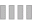# How To Choose Spring Rates For Your Car

To physically measure the front Motion Ratio, we then removed the front springs so that we could stoke the suspension, meaning move it through its range of motion without the resistance the springs normally provide. To measure Motion Ratio this way, we put a cable tie on the strut piston and took a measurement from the hub to the floor and then compressed the suspension 1” and measured to see how far the zip tie was pushed up the piston shaft. We saw just shy of 1” at the strut when stroking the suspension upward 1” and saw the same at 2” and 3” of travel, which confirmed that the front Motion Ratio is just shy of 1:1 and that it remains consistent throughout the front suspension’s range of travel.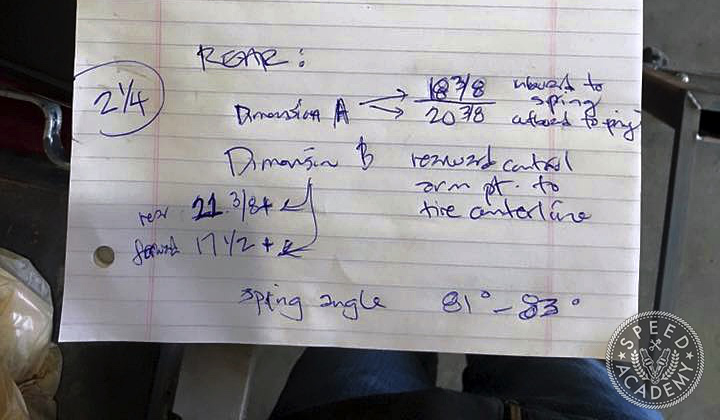Happy with our front Motion Ratio calculations and measurements, we moved on to the back, which was a bit more complicated given the spring has been moved to a coilover setup on the rear shocks instead of on the semi-trailing arm like it is when stock. Treating the inboard pivot axis of the semi-trailing arm as the starting point for both Motion Ratio measurements, we measured out to the centerline of the spring for Dimension A and to the centerline of rear tire contact patch to get Dimension B. Crunching the numbers we ended up with a rear Motion Ratio of 0.82. However, when we do the math with the spring perch on the semi-trailing arm the motion ratio was 0.62, so we were left scratching our heads a bit and hoping the stroking method would give us some clarity.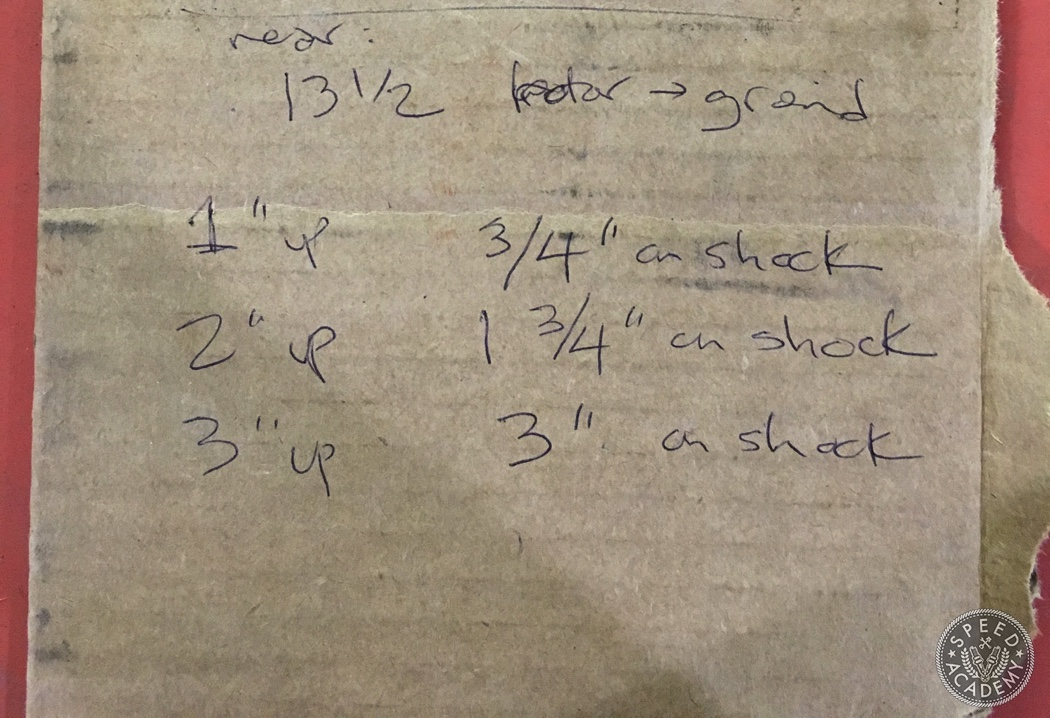We learned some interesting things here, starting with the fact that the Motion Ratio changes significantly as the suspension moves through its range of travel. As you can see, at 1” of travel we saw 3/4” of compression of the rear shock, which indicates a Motion Ratio of 0.75. However, the Motion Ratio increases to 0.875 at 2” of travel and 1.00 at 3” of travel. With an average Motion Ratio of 0.875 based on these three measurement points, we’re pretty close to our calculated Motion Ratio of 0.82 as measured at the coilover location.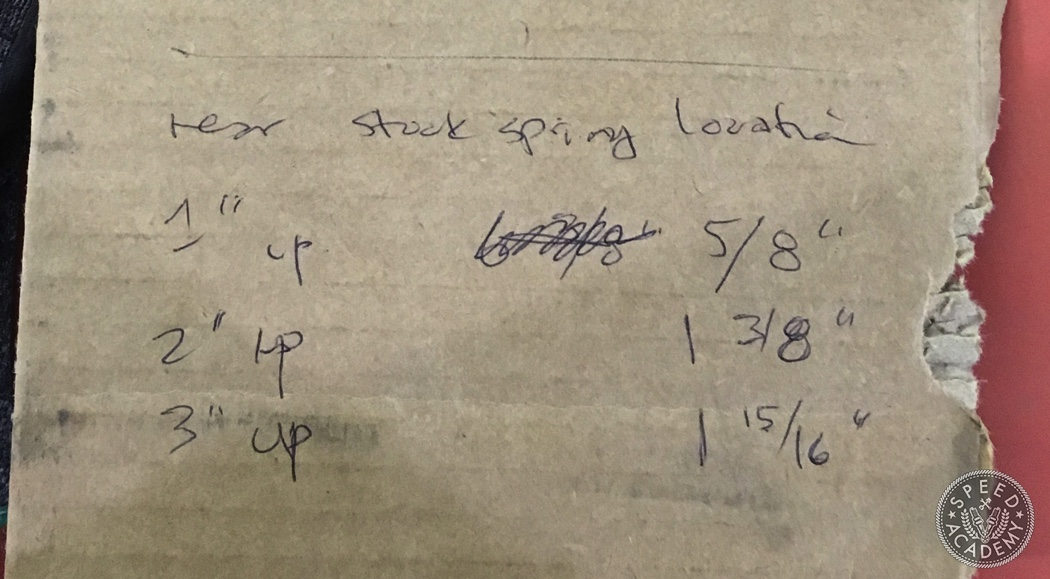However, when we measured suspension travel at the stock spring location on the rear semi-trailing arm, we saw very different results. As you can see, Motion Ratio at this location starts at 0.625 and increases to .6875 at 2” of travel and then decreases to 0.6458 at 3” of travel. So at the stock spring location, Motion Ratio appears to be significantly lower with an average around 0.65, which coincides closely with the ratio we calculated using the MR equation method.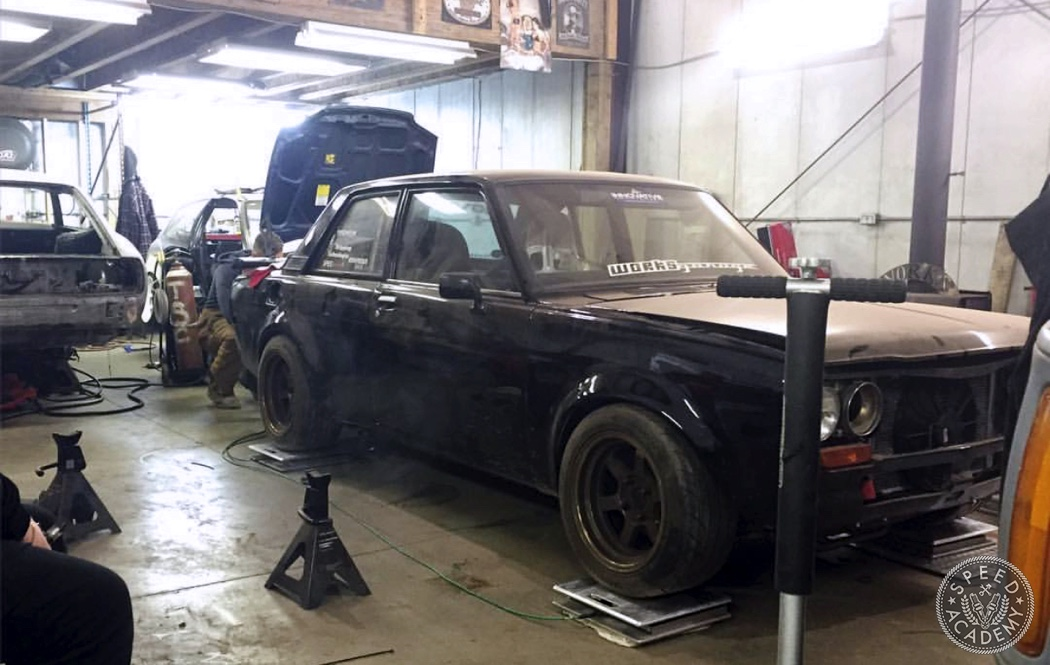Next, we needed to figure out the front and rear Wheel Rates, which meant JP had some weighing to do since determining Wheel Rate requires us to know the Sprung Weight of the car, which is most easily calculated by taking a total weight (on corner weight scales, which JP borrowed from Eric at R-Division, a local race shop) and then weighing the Unsprung components from a front and rear corner of the car. Here’s the Wheel Rate equation we used:

## WR = (SF/187.8)²(SW)

where SW is Sprung Weight and SF is Suspension Frequency (in cpm)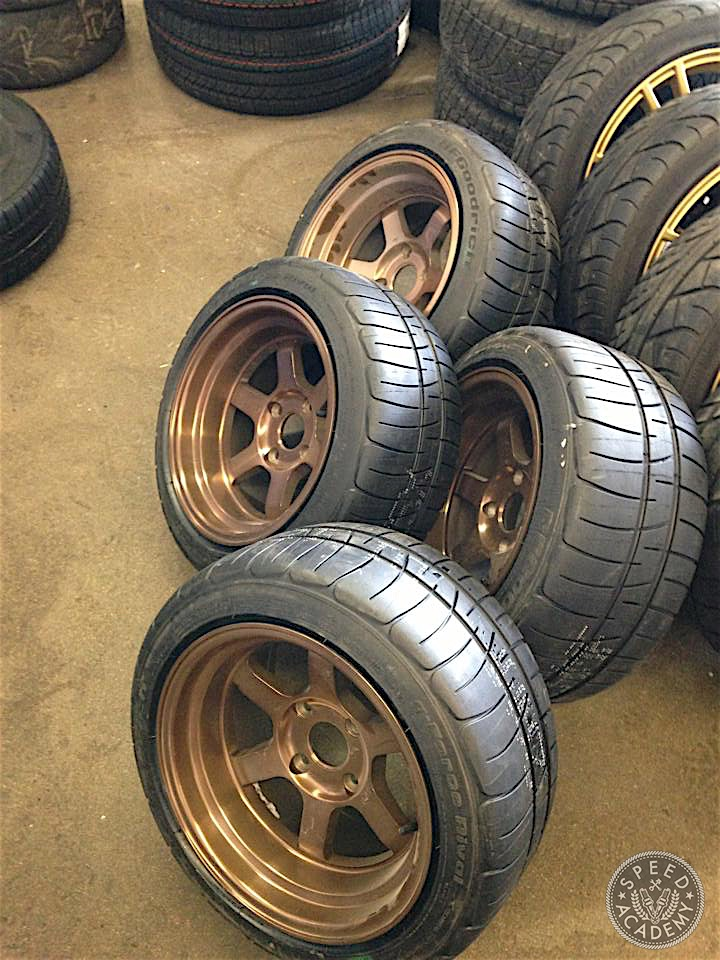Unsprung weight consists of all parts outboard from the shocks and springs, which includes the wheels, tires, brakes, steering knuckles, hubs, wheel bearings and wheel studs (anything that moves with the wheel as it travels over a bump is unsprung). Typically 50% of the weight of the axles, shocks, springs, and suspension links/arms is also included when calculating unsprung mass. On the front of the 510 we have the following unsprung components to account for when calculating unsprung mass: 15×8” wheels with 225/45-15 BFG Rivals @ 36 lbs, S14 calipers @ 9.5 lbs, S14 rotors @ 12.5 lbs, the S14 spindle, hub and lug nuts @ 17-lbs, and then 50% of the control arm, shock and spring @ 11 lbs for a grand total of 86 lbs. On the rear, we have to account for the hub @ 10 lbs, the wheel and tire combo @ 36 lbs, caliper @ 5 lbs, rotor at 9 lbs, and 50% of the axle, shock, spring and semi-trailing arm at 21 lbs for a total of 81 lbs.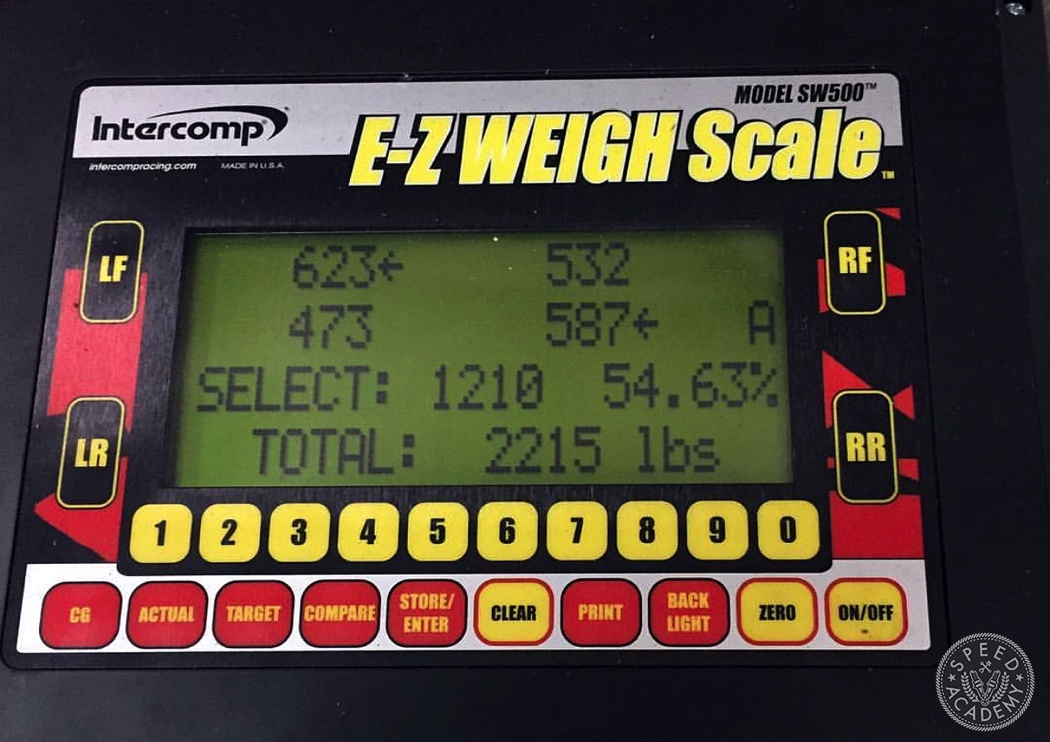With the above corner weights for JP’s 510, we can now calculate Wheel Rate for a given Suspension Frequency at each corner of the car. For example, the Sprung Weight of the front left (driver’s side) corner has a total weight of 623 lbs, so when we subtract 86 lbs for unsprung weight we’re left with a Sprung Weight of 537 lbs.

2 Comments on "How To Choose Spring Rates For Your Car"

Notify ofGuest

Having read an awful lot of opinions on the subject of spring angle correction factor, the consensus of opinion is that it should also be squared in the calculation, just like the motion ratio is. Any thoughts?Guest

When you think about it, you have already accounted for the spring angle on the front suspension with your empirical measurements because you measured the actual displacement of the strut in situ, ie at its installed angle. This measurement takes full account of the impact of both lever arm ratios and installed spring angle, on the motion ratio. If you wanted to apply the spring angle correction factor separately you should have measured only purely vertical displacement of ball joint on the suspension arm. This would have given only the lever arm motion ratio.